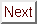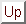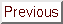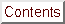Next: Discussion Up: Magnetotransport Measurements on Previous: Magnetoresistance Measurements: Experimental

## Results

Similar Shubnikov-de Haas oscillations were seen in all pure alpha-phase samples studied. Only very weak oscillations were seen in mixed-phase alpha + beta samples, probably due to a smaller value of (Omegac tau) caused by in-plane disorder. A representative Fourier transform calculated from the alpha-phase SdH data is shown in Figure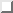a). This scan shows the frequency which was seen consistently, at (1120 ± 110) T.

In order to verify the authenticity of the highest frequency, the one used in the subsequent data analysis, various small portions of the data were Fourier transformed separately, to see if the 1120 T requency was an artifact of the analysis. However, it was found that this frequency appeared strongly no matter what field range of data was used for the Fourier transform. In addition, in order to get some intuition as to what the 1120 T frequency should look like experimentally, a calculated version of the trace was compared to the data using the fit parameters. The calculation used the accepted form of the temperature and field dependence of the amplitude of SdH oscillations:where A is the amplitude of the oscillations, TD is the Dingle temperature, and Omegac is the cyclotron frequency, through which A is dependent on the magnetic field. The Dingle temperature characterizes the degree of disorder in a solid through:where taucol is the transport collision time. An unusually large value of the Dingle temperature of about 5 K had to be used to produce the calculated trace shown in Figureb). The collision time in the C4CsBi0.6 samples is probably short due to the scattering from the metal inclusions discussed previously in Section. Confidence in the validity of the frequencies obtained from the Fourier transforms is increased because of the good qualitative agreement between the calculated curve and the data shown in Figureb).Figure: a) Fourier transform of the data in Figure. Most reproducible frequency is (1120 ± 110) T, although other frequencies sometimes occur. b) Comparison of the data from Figurewith a simulated trace calculated using Equationand parameters obtained from the Fourier transform of the data. The calculated curve is offset from the data for clarity.

Once the maximum SdH frequency of 1120 T has been determined, one can use the dilute-limit model as a first approach to understanding the data. This model assumes that the primary effect of intercalation is to increase the size of the graphitic pi-derived ellipsoids at the K point of the Brillouin zone, leaving the shape of the ellipsoids unmodified. The validity of this assumption for stage I GIC's could be criticized because of the high intercalant densities involved. However, the excellent agreement obtained by Timp et al. with other more direct measurements of Fermi energies and charge transfers gives some justification for the model's use. [These values are collected in Tablebelow.]

Proceeding then cautiously in the spirit of the Dresselhaus-Leung (DL) model, one can relate the compounds' Fermi energy to its maximal FS cross-section, cal Amaxe, and thus to the largest observed SdH frequency, numax. The expressions that describe this relationship, derived by DL, are:Here the E's are parameters of the DL model, the Gamma's are graphite band parameters, and a0 is the graphite in-plane lattice constant. Equationcan be used to solve for EF, giving a value of 0.93 eV. This value seems low for a stage 1 donor compound, as Tableshows. Yet, within the accuracy of the approximations made in the use of Equation, the agreement of this value with the 1.3 eV obtained by Yang and colleagues from reflectivity measurements is acceptable. The 1.3 eV number is itself based on the ``mirror bands'' approximation, whose quantitative correctness for donor compounds is not well-established.

With knowledge of the in-plane structure, one can also C4CsBi0.6 also estimate the charge transfer per carbon atom, fC. fC is approximately equal to the number of electrons per carbon atom in a GIC since the carrier density in pristine graphite is so low. The number of electrons per carbon atom is the ratio of the number of electrons per unit cell to the number of carbon atoms per unit cell. The number of electrons per unit cell is the ratio of the FS volume to the Brillouin zone (BZ) volume:

e- / C-atom = (e- /unit cell) / (C-atoms / unit cell)

The volume of a piece of pi-electron FS can be estimated in the spirit of the DL model as the size of a cylinder with a circular cross-section of area cal Amaxe and height 2pi / Ic:The total volume of the FS is then the number of cylinders per BZ (six) times the volume per cylinder. Note that the number of carbon atoms per unit cell and the volume of the BZ depend on the in-plane structure of the intercalant. At the time that Ref.  was published, the authors did not know of any work on the in-plane structural work on the CsBi-GIC's, and so calculated fC using the (2X2)R0°, since this structure is common in donor GIC's. A new number for fC has been calculated now that a specific in-plane structure has been observed in these materials.

Bendriss-Rerhrhaye in her thesis proposes a (3root13 X 8)R(15°,0°) rhombic unit cell for C4CsBi0.6. This proposed structure is shown in Figure. The BZ associated with such a unit cell will obviously be much smaller than the graphitic one, but this is partially compensated for by the large number of carbon atoms (192) in the unit cell. Using the 1120 T SdH frequency to calculate cal Amaxe gives an fC of -0.026 electrons per carbon atom. This value is about two-thirds of that obtained from reflectivity measurements by Yang et al., just as the EF obtained from SdH is about two-thirds of that from reflectivity. [See Table] The fC number of Yang and colleagues is obtained directly from their Fermi energy using the Blinowski-Rigaux (BR) tight-binding model. Therefore it is dependent on both the mirror-bands approximation (used to get EF) and the assumptions of the BR model, which, like the DL model, uses graphitic parameters to describe the pi bands of the intercalation compound. Taking all approximations into account, the EF and fC numbers of the two references should be about equally reliable. The lower value of the Fermi energy calculated from the SdH data could be due to a high-frequency oscillation that was not observed. A high-frequency oscillation appears to have been missed in the compound C4KHx. A frequency of about 1700 T would be needed for perfect agreement of the CsBi SdH data with the data of Yang.Figure: (3root13 X 8)R(15°,0°) in-plane unit cell proposed for C4CsBi0.6 by A. Bendriss-Rerhrhaye..Table: Electronic parameters for selected superconducting GIC's. The stage and phase of each compound are listed in parentheses. The compounds are listed in order of increasing superconducting transition temperature. Omegap is the unscreened plasma frequency.Next: Discussion Up: Magnetotransport Measurements on Previous: Magnetoresistance Measurements: Experimental

alchaiken@gmail.com (Alison Chaiken)
Wed Oct 11 22:59:57 PDT 1995